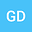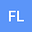Bifurcation for an overdetermined problem in the complement of a ball in $\mathbb{R}^N$
••We investigate the existence of a family nontrivial exterior domain $\tilde{\Omega}\subset \mathbb{R}^{N}$ $\left(N\geq2, N\neq3\right)$, bifurcating from the complement of a ball such that \begin{equation} \Delta u=0\,\, \text{in}\,\,\tilde{\Omega}, \,\, u=u_0,\,\,\partial_\nu u=\gamma H+C_0\,\,\text{on}\,\,\partial\tilde{\Omega},\,\,\,\lim _{r \rightarrow+\infty} u=0\,\, \text{or}\,\,+\infty\nonumber \end{equation} has a positive solution, where the Neumann condition is non-constant with $H$ is mean curvature and $\gamma$, $C_0$ are constants. This result gives a negative answer to the Berestycki-Caffarelli-Nirenberg conjecture on overdetermined elliptic problems in the complement of the ball.